﻿ Constrained-layer Damping Applied to DCJ Vibration Isolation DesignPublications are Open
Access in this journal
Article Versions
Export Article
Cite this article
• Normal Style
• MLA Style
• APA Style
• Chicago Style
Research Article
Open Access Peer-reviewed

### Constrained-layer Damping Applied to DCJ Vibration Isolation Design

G. M. Luo
Journal of Mechanical Design and Vibration. 2017, 5(1), 21-26. DOI: 10.12691/jmdv-5-1-3
Published online: May 11, 2017

### Abstract

A dual piezoelectric cooling jet (DCJ) is an innovative cooling device that uses piezoelectric materials to generate high-speed vibrations, thereby causing changes in the flow field to achieve heat exchange. Despite its high cooling efficiency, a DCJ transfers vibrations through its supporting base to its peripheral devices. To attenuate vibrations from DCJs, this study employed constrained-layer damping (CLD)-a technique for suppressing vibrations-to develop a base for cooling devices and to propose a C-DCJ model. ANSYS simulation of the vibrations of a DCJ and the C-DCJ suggested that, under the same vibration conditions and with the same levels of cooling efficiency, the amplitude and acceleration of the base on the C-DCJ were 30%–50% lower than that on the DCJ. Thus, the proposed C-DCJ effectively isolated vibration transfer.

### 1. Introduction

As precision machinery becomes increasingly miniaturized, numerous cooling devices such as dual piezoelectric cooling jets (DCJs) have been developed to replace conventional fans in such machines. A DCJ is a thin, highly efficient cooling device that uses piezoelectric materials to vibrate two thin metal sheets, thereby altering the flow of air to enable heat exchange. They are suitable to be employed in mobile phones and tablet computers.

Developed by General Electric in 2012, a DCJ has heat transfer efficiency 10 times greater than is achieved by natural convection and is inaudible to humans during operation 1. Research into this new cooling device began in 2013, when Sufian et al. 2 investigated the airflow and thermal distribution around a DCJ by conducting numerical analyses and making experimental measurements; the effects of the fan on transient-temperature distributions were identified and ABAQUS simulations suggested that the device was 2.3-times more efficient than a single-blade fan. The following year, Jang and Lee 3 performed a numerical analysis of the airflow characteristics of DCJs, altering the electric current to cause cyclic variations in the devices and allow them to continually produce airflow, and performed a spectral analysis of the airflow.

Using a DCJ to cool devices through heat transfer, however, causes additional vibrations. This limitation has posed a challenge to those seeking to improve the application of DCJs. Thus, this study used constrained-layer damping (CLD)-a technique for suppressing vibrations-to isolate vibrations from DCJs, and a C-DCJ model was proposed. ANSYS was employed to simulate the vibration of the C-DCJ model and a DCJ model and compare the difference of vibration between both models.

CLD involves the use of a viscoelastic gel between two thin metal sheets. The viscoelastic gel is restrained by the thin metal sheets during vibration, which causes additional shear deformation to dissipate energy; CLD can thus be used to dampen high-frequency vibrations. Moreover, the 0.4-mm-thick complex adds little volume to DCJs. Therefore, this study employed CLD in the vibration isolation design of its DCJ to dampen the vibrations caused by the DCJ.

Numerous studies have discussed the vibration characteristics of piezoelectric materials. Callahan et al. 4 used ultra-thin piezoelectric sheets to induce vibrations in cylindrical shells and conducted a modal analysis of the shells’ free vibration. Want 5 proposed a finite element model of bimorphs and performed static and dynamic analyses on them. Xu and Koko 6 examined the active control of smart structures through finite element analysis; used PZT-5H piezoelectric ceramics to control the vibration of cantilever beams; and conducted a finite-element-model analysis of the vibration of piezoelectric materials when they were placed at different positions. Furthermore, the vibration characteristics of piezoelectric materials have been extensively studied over the past decade. Nguyen et al. 7 attached piezoelectric sheets to aluminum cantilever beams to cause the beams to vibrate, simulated their vibration using ANSYS, and identified the relationship between vibration frequency and amplitude through harmonic analysis to determine suitable vibration frequencies. Dong et al. 8 placed a piezoelectric sheet on the upper and lower surfaces of a plate structure to generate a pure bending moment to suppress vibration; used SOLID45 to simulate metal materials and SOLID5 to simulate piezoelectric materials in ANSYS modeling; and conducted a transient analysis of the relationship between amplitude and time to investigate amplitude tapering. Sui et al. 9 installed a self-designed piezoelectric actuator on the support frame of an engine to counteract vibrations generated by the engine.

In addition to the aforementioned active vibration reduction mechanisms, damping materials are widely used to reduce the vibration of structures because they store and dissipate vibration energy. Their vibration-absorbing capability varies depending on their coefficients and their thickness. This study employed damping materials for vibration reduction on the basis of the following studies.

Choi et al. 10 proposed a shunted piezoelectric damping system to suppress multimodal vibrations. Malgaca 11 forced the vibration of smart laminated composites to actively control the materials and performed ANSYS simulations of vibration suppression using different damping materials that yielded results similar to the experimental results. Xie et al. 12 used CLD to suppress the vibration of rotating plates, indicating that thickening the CLD layer increased the deformation of its damping layer and accordingly improved its vibration reduction performance. Hong et al. 13 adjusted the damping of fiberglass reinforced plastic (FRP) propeller blades through lamination angle; proposed a method for predicting structural damping; and demonstrated that adjusting structural damping can effectively reduce blade vibration. Kaviani et al. 14 presented a vibration analysis of piezoelectric micropumps and used ANSYS to simulate the activation of the micropumps by piezoelectric materials under the effect of fluid damping. Zhong et al. 15 discussed the effect of shell vibration in microspeakers on the speaker’s output frequencies, using numerical simulations to demonstrate that retrofitting shells with damping materials can suppress noticeable audio distortions.

CLD is also used to solve vibration problems in mechanical structures. Oberst 16 used oscillatory differential equations to estimate the loss factor of free damping. Since Ross, Ungar, and Kerwin 17, 18 proposed the concept, CLD has been extensively employed. Ditaranto et al. 19, 20 investigated the heat-dissipation performance of CLD. Dowell et al. 21 derived equations governing the vibration of CLD plates and beams. Lu et al. 22 proposed a finite element model with damping to simulate the vibration of beams, plates, and rings and compared simulation and experimental results. John 23 conducted finite element analyses, deriving damping ratios and loss factors in different modes. Barkanov 24 used finite element analysis software to simulate the transient response of viscoelastic materials. Balamurugan et al. 25 elucidated the suppression and control of vibration using smart constrained layer damping (SCLD). Liu et al. 26 proposed active CLD and piezoelectrically induced active vibrations to enhance CLD performance, thereby inhibiting the vibration of a motherboard.

Numerous studies have focused on the application of CLD over the past 10 years. Zheng et al. 27 used a genetic algorithm to solve optimization problems involving a CLD structure, changing the position and length of the CLD structure to minimize vibrations in beams. Through finite element analysis and theoretical derivation, Luo 28, 29 examined the low-speed impact characteristics of FRP-laminated plates employing CLD and derived a vibration equation for the uniform impact load sustained by the CLD plates. Granger et al. 30 studied the transient vibration behavior on the millisecond timescale of beams partially employing CLD and discovered that the CLD attachment length exerted significant mitigating effects on the initial transient displacements of the beams, whereas the thickness of the viscoelastic layer had limited mitigating effects. Kumar et al. 31 used active/passive CLD to optimize the vibration performance of beams, suggesting that increasing the thickness and coverage area of a CLD structure can improve its damping performance. Lepoittevin et al. 32 segmented a CLD structure to enhance its damping performance, revealing that CLD segmentation may change the vibration mode and loss factor and doing so properly may improve its vibration reduction efficiency.

### 2. DCJ model

2.1. DCJ structure

The DCJ discussed in this study was characterized by two circular sheets of PZT (a widely used ceramic material) paste on two parallel 40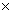40 mm2 and 0.11-mm-thin metal sheets (made of nickel iron alloy). Three of the edges of the metal sheets were sealed using 11 mm2 applications of viscoelastic gel, with the remaining edge unsealed to enable heat exchange.

DCJs drive airflow through the vibration of the PZT sheets, and this achieves heat exchange. The DCJ used in this study was suspended on its three sealed sides by viscoelastic gel on a base to prevent the transfer of vibrations from the PZT sheets to the base. The base comprised 1-mm-thick polyethylene (PE). The viscoelastic gel used to attach the base to the DCJ was 8 mm in length, 6 mm in width, and 1 mm in thickness. Figure 1 illustrates the structure of the DCJ.

• Figure 1. DCJ structure
2.2. Constrained-layer Damping

CLD was employed through a sandwich-type structure constructed by attaching viscoelastic gel and thin metal sheets to the base. The middle layer uses viscoelastic gel for damping and the outermost layers, or the restraint layers, are made of thin metal sheets to restrict the deformation of the gel. When a force is applied to the CLD sandwich, the gel undergoes shear deformation because of the restraint from the outermost layer, thereby dissipating the vibration energy. Figure 2 depicts the use of CLD.

• Figure 2. Constrained-layer damping

On the basis of 28, this study used Eq. (1) to describe the relationship between the shear stress and strain on the viscoelastic gel when it undergoes shear deformation. In this equation,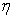is the loss factor and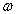is the natural vibration frequency.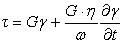(1)

The characteristics of viscoelastic gel are typically assessed by applying an exciting force, assuming that the system exhibits simple harmonic motion (SHM), and measuring the shear strain induced by vibration. In this case, the relationship between the shear stress and strain of the vibration can be expressed by Eq. (2), where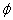is the phase difference due to the effect of damping on the vibration.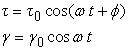(2)

Eq. (2) can be substituted into Eq. (1) to derive the shear stress–strain relationship when the viscoelastic gel is vibrated, as expressed by Eq. (3):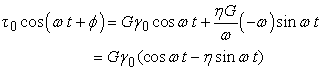(3)

This equation was simplified using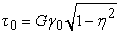, and the loss factor could be defined as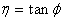, whereis related to the phase difference of the shear vibration. This phase difference is caused by the damping of the viscoelastic material;thus represents the damping of the material.

2.3. Finite Element Model

To elucidate the transfer of vibration from the DCJ and to develop a way to isolate it, this study performed numerical simulations using ANSYS and used solid elements to develop a finite element DCJ model in accordance with the actual characteristics and size of the DCJ displayed in Figure 1. In the model (Figure 3), the simulated thin metal sheets, viscoelastic gel, and base were based on SOLID45, whereas the simulated PZT was based on SOLID5.

• Figure 3. DCJ finite element model

An additional thin aluminum sheet was attached to the viscoelastic gel to restrict the deformation of the gel, completing the formation of a CLD structure needed to isolate the transfer of vibration from the DCJ model to the base. Figure 4 displays the DCJ model attached to the CLD structure, which was named C-DCJ in this study.

• Figure 4. C-DCJ finite element model
2.4. Material parameters

The viscoelastic gel used in the simulations was ISD-112 (3M). In the DCJ model, the two thin metal sheets were made of nickel iron alloy and the base material was PE. Table 1 lists the material parameters of the gel, base, and thin metal sheets. PZT-5H, 25 mm in diameter and 0.11 mm in thickness, was used as the PZT material. During ANSYS analysis of piezoelectric materials, the stiffness, stress, and dielectric matrices of the materials were calculated. Table 2 presents the material parameters of PZT-5H.

### 3. Discussion

3.1. Vibration perception

CLD was used to isolate the transfer of vibrations from the DCJ model to the base, and whether these vibrations could be perceived by humans was measured.

The amount of vibration perceived by humans was calculated in accordance with ISO 2631-1 33, wherein the root mean square (RMS) of the vibration acceleration was used to quantify the perceived comfort of vibrations, as expressed by Eq. (4):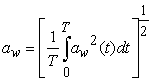(4)

Where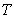is the measurement duration and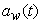is the time-dependent acceleration function, which can be specified as translational or rotational. Translational RMS acceleration was adopted because this study investigated perceptible vibrations transferred from the DCJ model to the base.Calculating the amplitude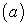and frequency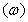of vibration allowto be determined, as expressed by Eq. (5):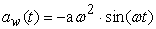(5)

A numerical simulation revealed the amplitude and frequency of vibration transferred to the base as a function of time, which were then substituted into Eq. (5) to derive, subsequently differentiated in Eq. (4) to yield the RMS acceleration of vibration.

According to ISO 2631-1 statistics, approximately half of adults can perceive vibrations of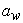= 0.015 m/s2. Although vibration perceptibility varies from person to person, the average range of perceptible vibrations is 0.01–0.02 m/s2. Accordingly, this study defined an RMS acceleration of 0.02 m/s2 as the threshold above which vibrations can be perceived.

3.2. Simulation Results

ANSYS was used to perform transient analyses simulating the vibration behavior of the DCJ model, with the frequency of vibration set to 150 Hz and the maximum amplitude of vibration in the middle of thin metal sheets set to less than 0.5 mm to prevent their collision during vibration. Regarding the specification of boundary conditions, the four holes on the base were assumed to be fixed and the amount of vibration at four measurement points (respectively labeled A, B, C, and D in Figure 5) was measured. Measure point A represented the amplitude of opening of two meatal sheets. Measurement Point B represented the amplitude of vibration of the PZT in the middle of the DCJ model, and Measurement Points C and D denoted the amplitude of vibration at two points on the base.

• Figure 5. Boundary conditions of DCJ

The damping of the viscoelastic materials was characterized through Rayleigh damping, in which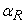is the damping generated by motion in the damping environment at each mass point and is related to mass; additionally,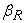is the damping of the viscoelastic materials. Given that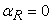,can be derived through the loss factor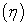and frequency of amplitude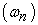; as such,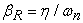. The loss factor of ISD-112 (the gel used) is 0.9 at a frequency of vibration of 150 Hz.

Figure 6 illustrates the mode of vibration of the DCJ model. The maximum amplitude of vibration occurred in the middle of and at the free boundary of the two thin metal sheets. Thus, the mode of vibration of the DCJ model was normal actuation. Under such vibration conditions, the amplitudes at the four measurement points were compared and the vibration-isolating performance of the CLD structure was determined.

• Figure 6. Vibration mode of DCJ

Additionally, the analysis revealed complete consistency in the amplitude and frequency of vibrations at Measurement Points A and B between the DCJ and C-DCJ models, indicating that the cooling efficiency of the DCJ model was not decreased by the use of CLD. Figure 7 and Figure 8 plot the amplitude of vibration as a function of time. At Measurement Point A, both models had a maximum initial amplitude of vibration of 0.34 mm and exhibited stable vibrations of amplitude 0.19 mm. At Measurement Point B, the models had a maximum initial amplitude of vibration of 0.45 mm and exhibited stable vibrations of amplitude 0.24 mm. The amplitude of vibration at both points was less than 0.5 mm, satisfying the requirement of initial amplitude of vibration. This avoided collision of the metal sheets.

Figure 9 depicts the amplitude of vibration at Measurement Point C versus time. The initial vibrations in the C-DCJ and DCJ models at Measurement Point C exhibited phase differences because of the CLD, but the models vibrated stably and in phase after this initial period. In particular, after the vibrations had become stable in the C-DCJ model, the amplitude of vibration was only 1.1 10−4 mm, compared with 1.6 10−4 mm in the DCJ model. This suggested that CLD adequately isolated vibrations, damping 31.25% of the vibration at Measurement Point C.

The most substantial vibration isolation occurred at Measurement Point D (Figure 10). The maximum amplitude of vibration in the C-DCJ model at Measurement Point D was 4.4 104 mm, far lower than that discovered in the DCJ model (8.9 10−4 mm). Thus, the vibration isolation efficiency of the C-DCJ model was 50.56%. CLD thus significantly reduced the vibration of the DCJ. Table 3 summarizes the data of the stable vibrations at all measurement points.

• Figure 7. Amplitude of measure point A
• Figure 8. Amplitude of measure point B
• Figure 9. Amplitude of measure point C
• Figure 10. Amplitude of measure point D

In summary, the transfer of vibrations from the C-DCJ to the base was effectively isolated without decreasing the DCJ’s cooling efficiency. This study also determined whether the estimated vibrations could be perceived by humans in accordance with ISO 2631-1.

The minimum RMS acceleration that can be perceived by humans was set as 0.02 m/s2. The frequency and amplitude of vibrations at Measurement Points C and D were estimated according to ISO 2631-1 and the RMS acceleration at both points was determined, as summarized in Table 4. The RMS acceleration at these points in the DCJ model was greater than 0.02 m/s2 and thus could be perceived by humans. The RMS acceleration at Measurement Point C in the C-DCJ model had the critical value where vibration could not be perceived by everybody, whereas that at Measurement Point D was within the perceptible range and approximately 50% lower than at the same point in the DCJ model, indicating a noticeable reduction in vibration.

### 4. Conclusion

This study proposed a design solution to isolating the transfer of vibrations from DCJs to their base. The DCJ model used in this study had its thin metal sheets attached by viscoelastic gel to the base. An ultra-thin aluminum sheet was attached to the gel to form a CLD structure in which vibrations induced shear deformation in the gel, causing its vibration and the dissipation of energy, thereby effectively isolating the transfer of vibration from the thin metal sheets to the base without compromising the operational efficiency of the DCJ model.

The results of numerical simulations suggested that the C-DCJ model isolated the transfer of its vibration effectively, reduced the maximum amplitude of vibration following isolation and the RMS velocities associated with vibration by 30%–50%, and decreased the acceleration of vibration in some regions of the base such that it would be imperceptible. These findings on the remarkable ability of CLD to isolate vibrations may inform the design of DCJ vibration reduction and isolation.

### References

  H. Peter de Bocka, P. Chamarthya, J. L. Jacksonb, B. Whalena, Investigation and application of an advanced dual piezoelectric cooling jet to a typical electronics cooling configuration. Thermal and Thermomechanical Phenomena in Electronic Systems, 2012 13th IEEE Intersociety Conference, 1387-1394, San Diego, CA, IEEE. In article View Article  S. F. Sufian, M. Z. Abdullah, J. J. Mohamed, Effect of synchronized piezoelectric fans on microelectronic cooling performance. International Communications in Heat and Mass Transfer, 43, 81-89, 2013. In article View Article  D. Jang, K. Lee, Flow characteristics of dual piezoelectric cooling jets for cooling applications in ultra-slim electronics. International Journal of Heat Mass Transfer, 79, 201-211, 2014. In article View Article  J. Callahan, H. Baruh, Vibration monitoring of cylindrical shells using piezoelectric sensors. Finite Elements in Analysis Design, 23, 303-318, 1996. In article View Article  S. Y. Wang, A finite element model for the static and dynamic analysis of a piezoelectric bimorph. International Journal of Solids and Structures, 41, 4075-4096, 2004. In article View Article  S. X. Xu, T. S. Koko, Finite element analysis and design of actively controlled piezoelectric smart structures. Finite Elements in Analysis Design, 40, 241-262, 2004. In article View Article  C. H. Nguyen, S. J. Pietrzko, FE analysis of a PZT-actuated adaptive beam with vibration damping using a parallel R–L shunt circuit. Finite Elements in Analysis Design, 42, 1231-1239, 2006. In article View Article  X. J. Dong, G. Meng, J. C. Peng, Vibration control of piezoelectric smart structures based on system identification technique: Numerical simulation and experimental study. Journal of Sound and Vibration, 297, 680-693, 2006. In article View Article  L. Sui, X. Xiong, G. Shi, Piezoelectric actuator design and application on active vibration control. Physics Procedia, 25, 1388-1396, 2012. In article View Article  S. B. Choi, H. S. Kim, J. S. Park, Multi-mode vibration reduction of a CD-ROM drive base using a piezoelectric shunt circuit. Journal of Sound and Vibration, 300, 160-175, 2007. In article View Article  L. Malgaca, Integration of active vibration control methods with finite element models of smart laminated composite structures. Composite Structures, 92, 1651-1663, 2010. In article View Article  Z. Xie, X. Xue, A new plate finite element model for rotating plate structures with constrained damping layer. Finite Elements in Analysis and Design, 47, 487-495, 2011. In article View Article  Y. Hong, X. D. He, R. G. Wang, Vibration and damping analysis of a composite blade. Materials & Design, 34, 98-105, 2012. In article View Article  S. Kaviani, M. Bahrami, A. M. Esfahani, B. Parsi, A modeling and vibration analysis of a piezoelectric micro-pump diaphragm. Comptes Rendus Mécanique , 342, 692-699, 2014. In article View Article  X. Zhong, Q. Wu, X. Li, Influence of enclosure wall vibration on the frequency response of miniature loudspeakers. Applied Acoustics, 93, 9-14, 2015. In article View Article  H. Oberst, Uber die Damping der Biegeschwingungen dunner Bleche durch fest haftende Belage. Acta Acustica united with Acustica, 2, 181-194, 1952. In article  R. Ross, E. E. Ungar, E. M. Kerwin, Damping of plate flexural vibration by means of viscoelastic laminate. Structural Damping, ASME, New York, 1959. In article View Article  E. M. Kerwin, Damping of flexural waves by a constrained viscoelastic layer. Acoustical Society of America, 31, 952-965, 1959. In article View Article  R. A. Ditaranto, Theory of vibratory bending for elastic and viscoelastic layer. Applied Mechanics, 32, 881-886, 1965. In article View Article  R. A. Ditaranto, J. R. McGraw, Vibratory damping for laminated plates. Engineering for Industry, 91, 1081-1090, 1969. In article View Article  M. J. Yan, E. H. Dowell, Governing equations for vibrating constrained layer damping sandwich plates and beams. Applied Mechanics, 39, 1041-1046, 1972. In article View Article  Y. P. Lu, G. C. Everstine, More on finite element modeling of damped composite systems. Sound and Vibration, 69, 199-205, 1980. In article View Article  C. D. Johnson, D. A. Kienholz, Finite element prediction of damping in structures with constrained viscoelastic layers. AIAA Journal, 20, 1284-1290, 1982. In article View Article  E. Barkanov, Transient response analysis of structures made form viscoelastic materials. International journal for numerical methods in engineering, 44, 393-403, 1999. In article View Article  V. Balamurugan, S. Narayanan, Finite Element Formulation and Active Vibration Control Study on Beams Using Smart Constrained Layer Damping (SCLD) Treatment. Journal of Sound and Vibration, 249, 227-250, 2002. In article View Article  T. X. Liu, H. X. Hua, Z. Zhang, Robust control of plate vibration via active constrained layer damping. Thin-Walled Structures, 42, 427-448, 2004. In article View Article  H. Zheng, G. S .H. Pau, Y. Y. Wang, A comparative study on optimization of constrained layer damping treatment for structural vibration control. Thin-Walled Structures, 44, 886-896, 2006. In article View Article  G. M. Luo, Y. J. Lee, Simulation of constrained layered damped laminated plates subjected to low-velocity impact using a quasi-static method. Composite Structures, 88, 290-295, 2009. In article View Article  G. M. Luo, Y. J. Lee, C. H. Huang, The application and conduct of vibration equations for constrained layered damped plates with impact. J. Steel and Composite Structures, 8, 4, 281-296, 2008. In article View Article  D. Granger, A. Ross, Effects of partial constrained viscoelastic layer damping parameters on the initial transient response of impacted cantilever beams: Experimental and numerical results. Journal of Sound and Vibration, 321, 45-64, 2009. In article View Article  N. Kumar, S. P. Singh, Vibration and damping characteristics of beams with active constrained layer treatments under parametric variations. Materials & Design, 30, 4162-4174, 2009. In article View Article  G. Lepoittevin, G. Kress, Optimization of segmented constrained layer damping with mathematical programming using strain energy analysis and modal data. Materials & Design, 31, 14-24, 2010. In article View Article  ISO 2631-1-1997: Mechanical vibration and shock-Evaluation of human exposure to whole-body vibration-Part1 General requirements. In articleThis work is licensed under a Creative Commons Attribution 4.0 International License. To view a copy of this license, visit http://creativecommons.org/licenses/by/4.0/

### Cite this article:

##### Normal Style
G. M. Luo. Constrained-layer Damping Applied to DCJ Vibration Isolation Design. Journal of Mechanical Design and Vibration. Vol. 5, No. 1, 2017, pp 21-26. http://pubs.sciepub.com/jmdv/5/1/3
##### MLA Style
Luo, G. M.. "Constrained-layer Damping Applied to DCJ Vibration Isolation Design." Journal of Mechanical Design and Vibration 5.1 (2017): 21-26.
##### APA Style
Luo, G. M. (2017). Constrained-layer Damping Applied to DCJ Vibration Isolation Design. Journal of Mechanical Design and Vibration, 5(1), 21-26.
##### Chicago Style
Luo, G. M.. "Constrained-layer Damping Applied to DCJ Vibration Isolation Design." Journal of Mechanical Design and Vibration 5, no. 1 (2017): 21-26.
Share